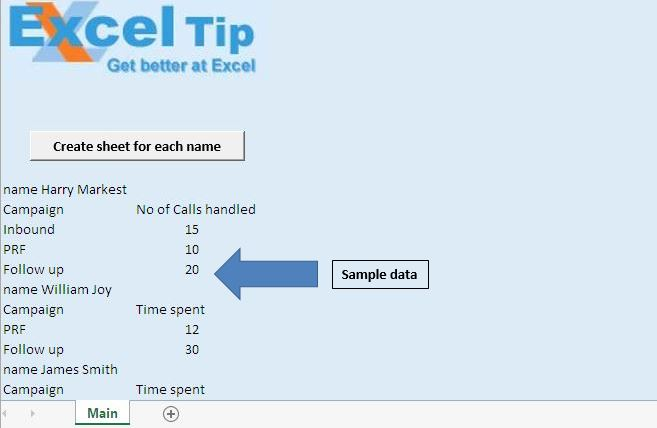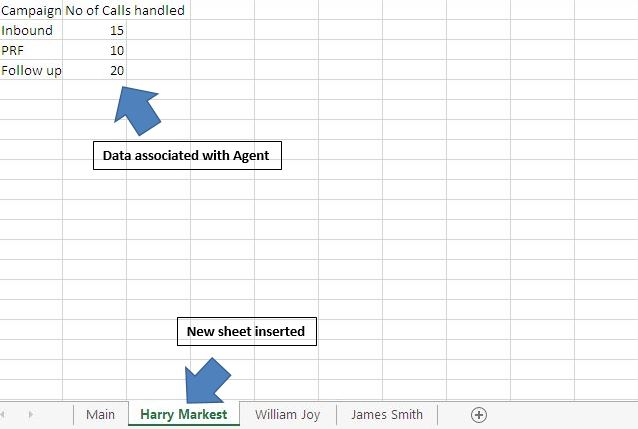# Create separate worksheets for each name mentioned in the data using VBA in Microsoft Excel.

In this article, we will create a macro to create separate worksheets for each name mentioned in the data.

Raw data consists of Name followed by Campaign details and Number of calls handled.In this example, we want to create separate worksheets for each name and the sheet will be having data related to campaigns and number of calls handled by agent.Logic explanation

In this article, we have created “AfterNamesCopying” macro. It will separate the data to different sheets, based on agent name. To separate the data we check for “name” in data and copy the data below “name” row to the respective sheet.

Code explanation

Left(WksData.Cells(IntRow, 1), 4) = "name"

The above code is used to check whether value in a cell begins with “name”.

Right(WksData.Cells(IntRow, 1), Len(WksData.Cells(IntRow, 1)) – 5)

The above code is used to extract agent name from the cell value.

The above code is used to insert a new worksheet, after the last worksheet.

ActiveSheet.Name = StrSheet

The above code is used to rename the active sheet.

Range(.Cells(IntRowL, 1), .Cells(IntRowL, 3)).Value = _

Range(WksData.Cells(IntRow, 1), WksData.Cells(IntRow, 3)).Value

The above code is used to add data related to that particular agent.

```Option Explicit

Sub AfterNamesCopying()

'Declaring variables
Dim wks As Worksheet, WksData As Worksheet
Dim IntRow As Integer, IntRowL As Integer
Dim StrSheet As String

Application.ScreenUpdating = False

'Initializing variables
Set WksData = ActiveSheet
IntRow = 10

'Loop until cell in first column is empty
Do Until IsEmpty(WksData.Cells(IntRow, 1))

'Checking whether value in the cell begins with string "name"
If Left(WksData.Cells(IntRow, 1), 4) = "name" Then

'Extracting name from the cell value
StrSheet = Right(WksData.Cells(IntRow, 1), Len(WksData.Cells(IntRow, 1)) - 5)

'Renaming the sheet
ActiveSheet.Name = StrSheet
IntRowL = 1

Else

With Worksheets(StrSheet)
'Inserting data to respective sheets
Range(.Cells(IntRowL, 1), .Cells(IntRowL, 3)).Value = _
Range(WksData.Cells(IntRow, 1), WksData.Cells(IntRow, 3)).Value
End With
IntRowL = IntRowL + 1
End If

IntRow = IntRow + 1
Loop

Application.ScreenUpdating = True

End Sub
```